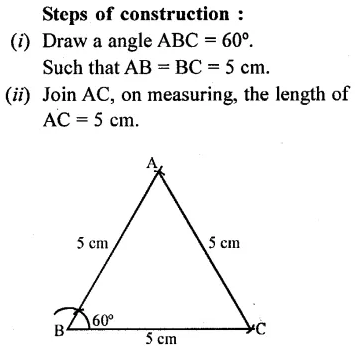# Selina Concise Mathematics Class 6 ICSE Solutions Chapter 25 Properties of Angles and Lines

## Selina Concise Mathematics Class 6 ICSE Solutions Chapter 25 Properties of Angles and Lines (Including Parallel Lines)

Selina Publishers Concise Mathematics Class 6 ICSE Solutions Chapter 25 Properties of Angles and Lines (Including Parallel Lines)

### Properties of Angles and Lines Exercise 25A – Selina Concise Mathematics Class 6 ICSE Solutions

Question 1.
Two straight lines AB and CD intersect each other at a point O and angle AOC = 50° ; find :
(i) angle BOD
(ii) ∠AOD
(iii) ∠BOC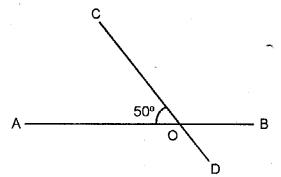Solution:Question 2.
The adjoining figure, shows two straight lines AB and CD intersecting at point P. If ∠BPC = 4x – 5° and ∠APD = 3x + 15° ; find :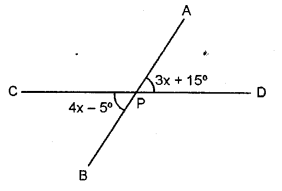(i) the value of x.
(ii) ∠APD
(iii) ∠BPD
(iv) ∠BPC
Solution:Question 3.
The given diagram, shows two adjacent angles AOB and AOC, whose exterior sides are along the same straight line. Find the value of x.Solution: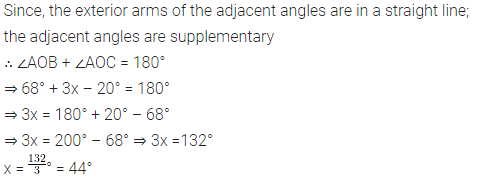Question 4.
Each figure given below shows a pair of adjacent angles AOB and BOC. Find whether or not the exterior arms OA and OC are in the same straight line.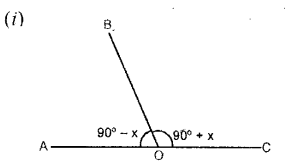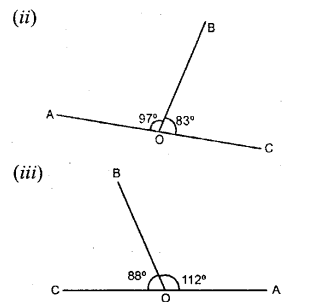Solution:Question 5.
A line segment AP stands at point P of a straight line BC such that ∠APB = 5x – 40° and ∠APC = .x+ 10°; find the value of x and angle APB.
Solution:### Properties of Angles and Lines Exercise 25B – Selina Concise Mathematics Class 6 ICSE Solutions

Question 1.
Identify the pair of angles in each of the figure given below :
adjacent angles, vertically opposite angles, interior alternate angles, corresponding angles or exterior alternate angles.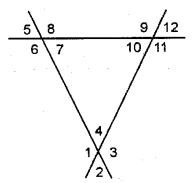Solution: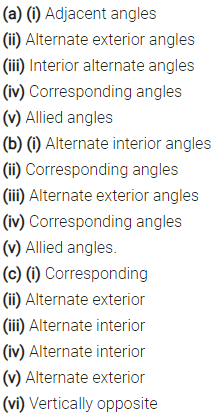Question 2.
Each figure given below shows a pair of parallel lines cut by a transversal For each case, find a and b, giving reasons.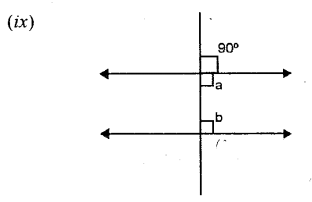Solution: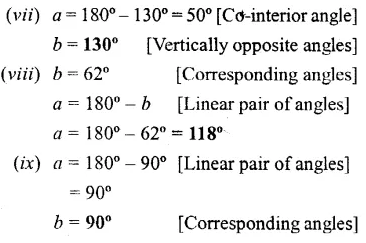Question 3.
If ∠1 = 120°, find the measures of : ∠2, ∠3, ∠4, ∠5, ∠6, ∠7 and ∠8. Give reasons.Solution:Question 4.
In the figure given below, find the measure of the angles denoted by x,y, z,p,q and r.Solution:Question 5.
Using the given figure, fill in the blanks.Solution:Question 6.
In the given figure, find the anlges shown by x,y, z and w. Give reasons.Solution:Question 7.
Find a, b, c and d in the figure given below :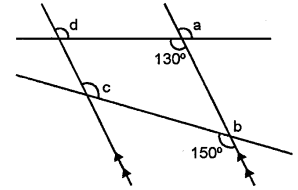Solution: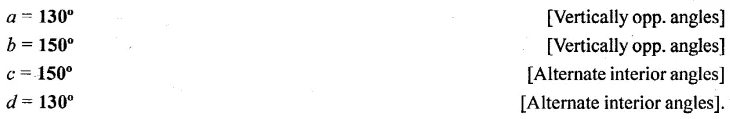Question 8.
Find x, y and z in the figure given below :Solution:### Properties of Angles and Lines Exercise 25C – Selina Concise Mathematics Class 6 ICSE Solutions

Question 1.
In your note-book copy the following angles using ruler and a pair compass only.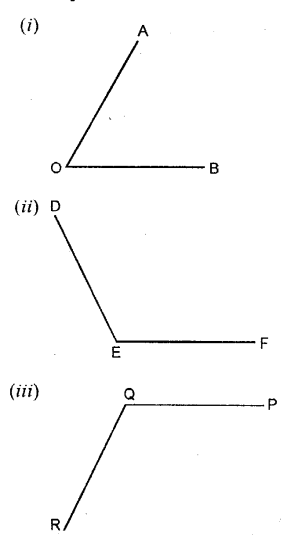Solution: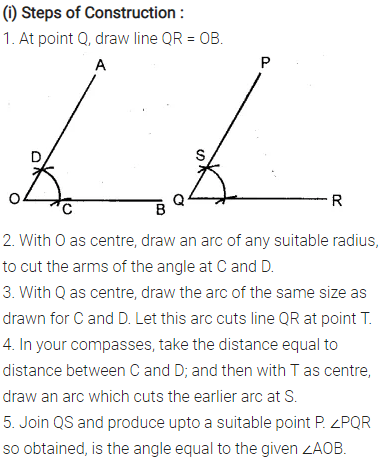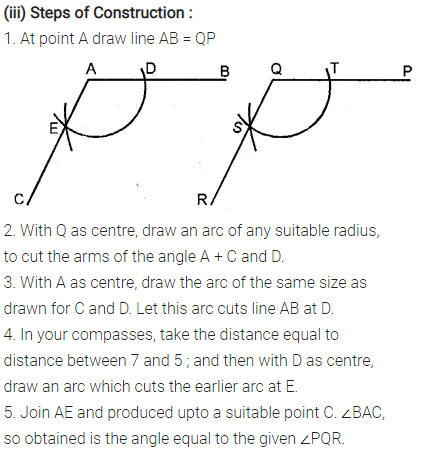Question 2.
Construct the following angles, using ruler and a pair of compass only
(i) 60°
(ii) 90°
(iii) 45°
(iv) 30°
(v) 120°
(vi) 135°
(vii) 15°
Solution: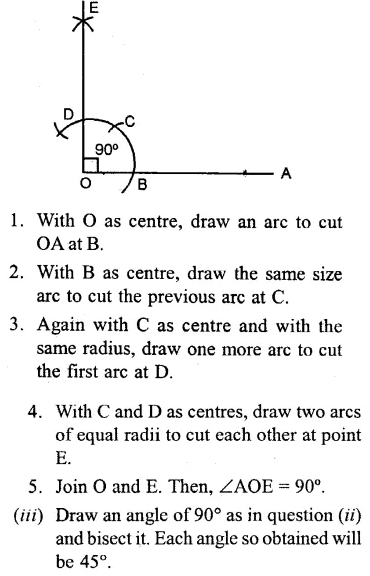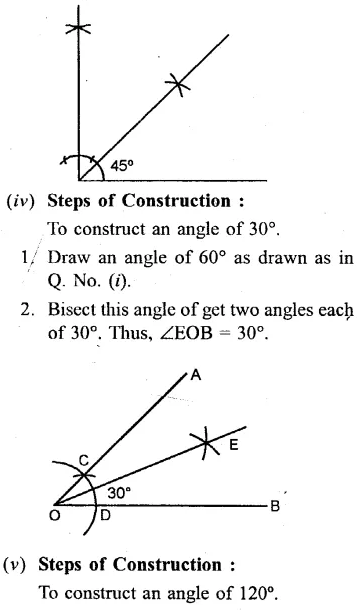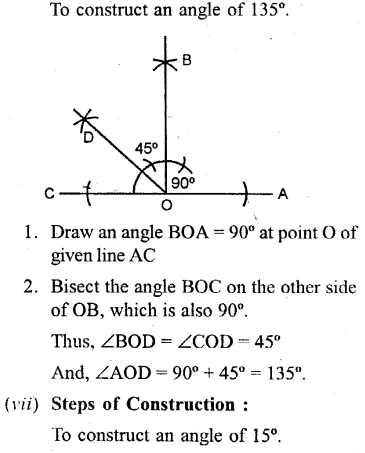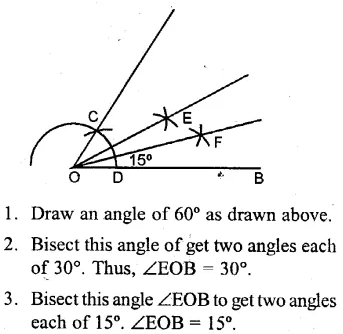Question 3.
Draw line AB = 6cm. Construct angle ABC = 60°. Then draw the bisector of angle ABC.
Solution:Question 4.
Draw a line segment PQ = 8cm. Construct the perpendicular bisector of the line segment PQ. Let the perpendicular bisector drawn meet PQ at point R. Measure the lengths of PR and QR. Is PR = QR ?
Solution: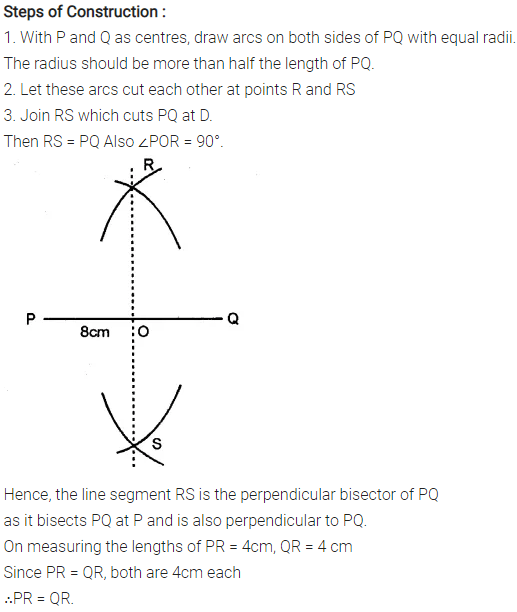Question 5.
Draw a line segment AB = 7cm. Mark a point Pon AB such that AP=3 cm. Draw perpendicular on to AB at point P.
Solution:Question 6.
Draw a line segment AB = 6.5 cm. Locate a point P that is 5 cm from A and 4.6 cm from B. Through the point P, draw a perpendicular on to the line segment AB.
Solution: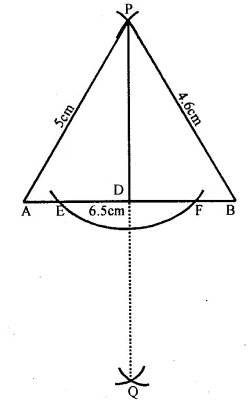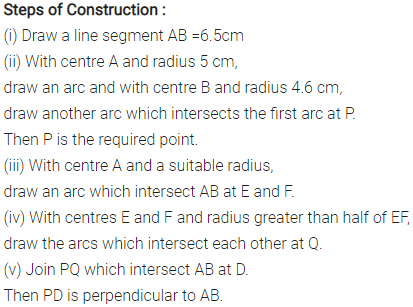### Properties of Angles and Lines Exercise 25D – Selina Concise Mathematics Class 6 ICSE Solutions

Question 1.
Draw a line segment OA = 5 cm. Use set-square to construct angle AOB = 60°, such that OB = 3 cm. Join A and B ; then measure the length ofAB.
Solution: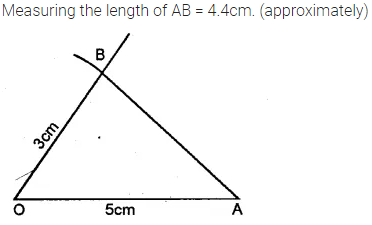Question 2.
Draw a line segment OP = 8cm. Use set-square to construct ∠POQ = 90°; such that OQ = 6 cm. Join P and Q; then measure the length of PQ.
Solution:Question 3.
Draw ∠ABC = 120°. Bisect the angle using ruler and compasses. Measure each angle so obtained and check whether or not the new angles obtained on bisecting ∠ABC are equal.
Solution: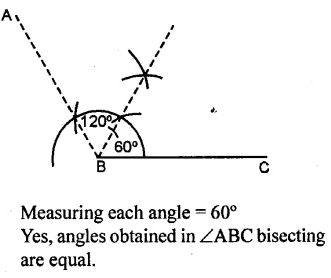Question 4.
Draw ∠PQR = 75° by using set- squares. On PQ mark a point M such that MQ = 3 cm. On QR mark a point N such that QN = 4 cm. Join M and N. Measure the length of MN.
Solution:### Properties of Angles and Lines Revision Exercise – Selina Concise Mathematics Class 6 ICSE Solutions

Question 1.
In the following figures, AB is parallel to CD; find the values of angles x, y and z :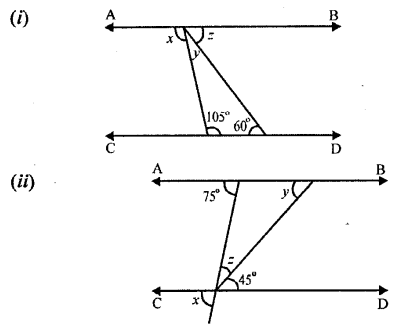Solution: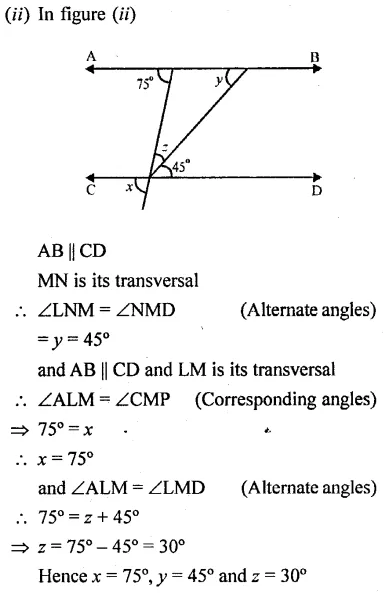Question 2.
In each of the following figures, BA is parallel to CD. Find the angles a, b and c:Solution: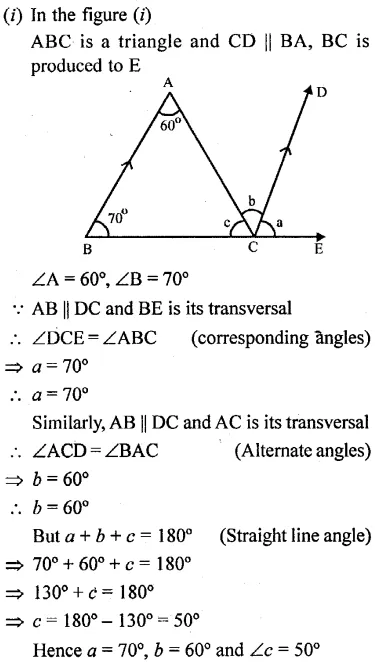Question 3.
In each of the following figures, PQ is parallel to RS. Find the angles a, b and c: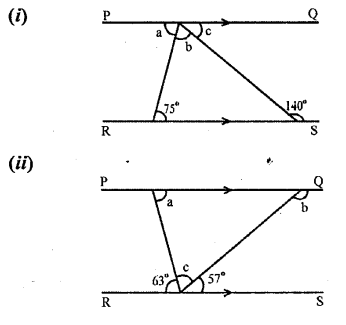Solution: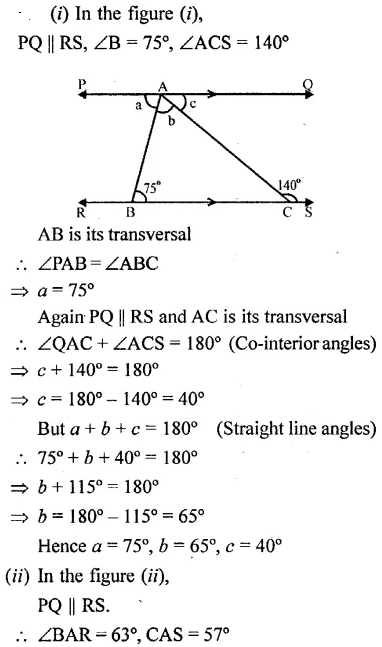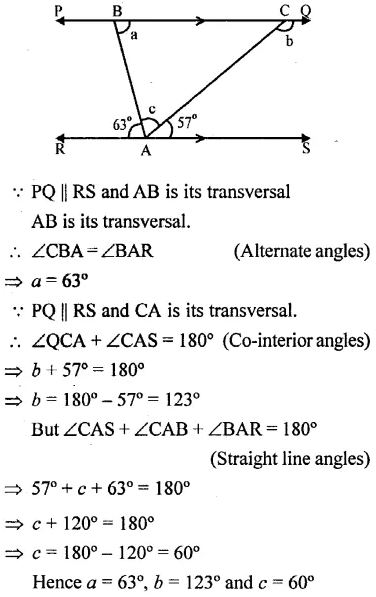Question 4.
Two straight lines are cut by a transversal. Are the corresponding angles always equal?
Solution: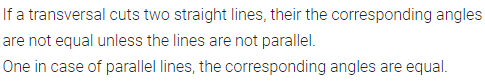Question 5.
Two straight lines are cut by a transversal so that the co-interior angles are supplementary. Are the straight lines parallel?
Solution: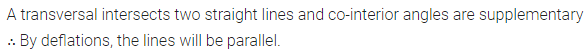Question 6.
Two straight lines are cut by a transversal so that the co-interior angles are equal. What must be the measure of each interior angle to make the straight lines parallel to each other ?
Solution: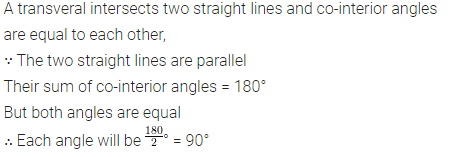Question 7.
In each case given below, find the value of x so that POQ is straight line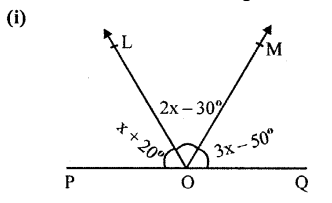Solution: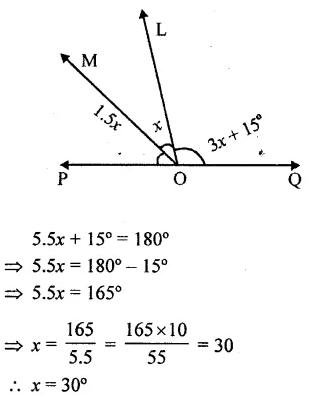Question 8.
in each case, given below, draw perpendicular to AB from an exterior point P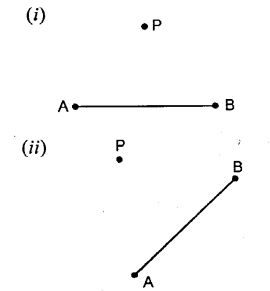Solution: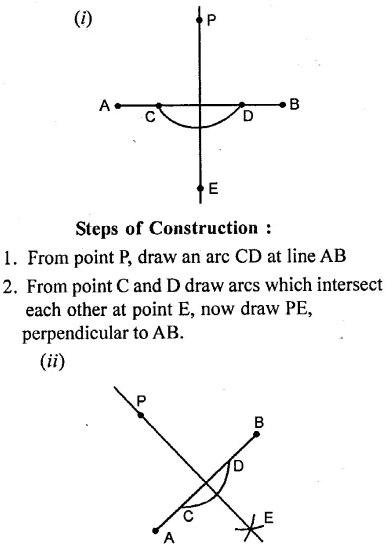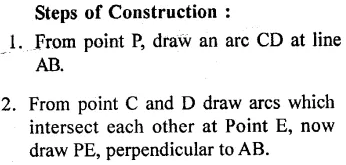Question 9.
Draw a line segment BC = 8 cm. Using set-squares, draw ∠CBA = 60° and ∠BCA = 75°. Measure the angle BAC. Also measure the lengths of AB and AC.
Solution: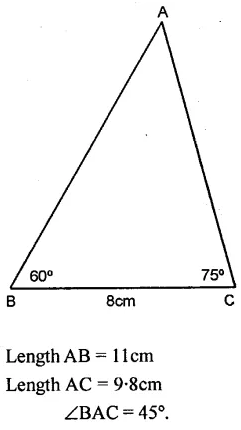Question 10.
Draw a line AB = 9 cm. Mark a point P in AB such that AP=5 cm. Through P draw (using set-square) perpendicular PQ = 3 cm. Measure BQ.
Solution: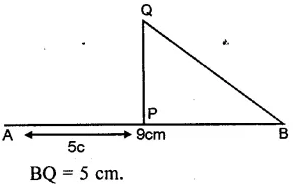Question 11.
Draw a line segment AB = 6 cm. Without using set squares, draw angle OAB = 60° and angle OBA = 90°. Measure angle AOB and write this measurement.
Solution: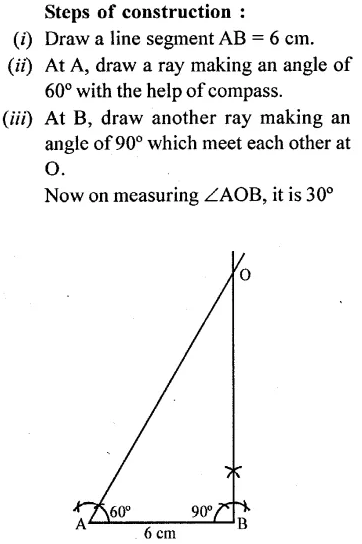Question 12.
Without using set squares, construct angle ABC = 60° in which AB = BC = 5 cm. Join A and C and measure the length of AC.
Solution: